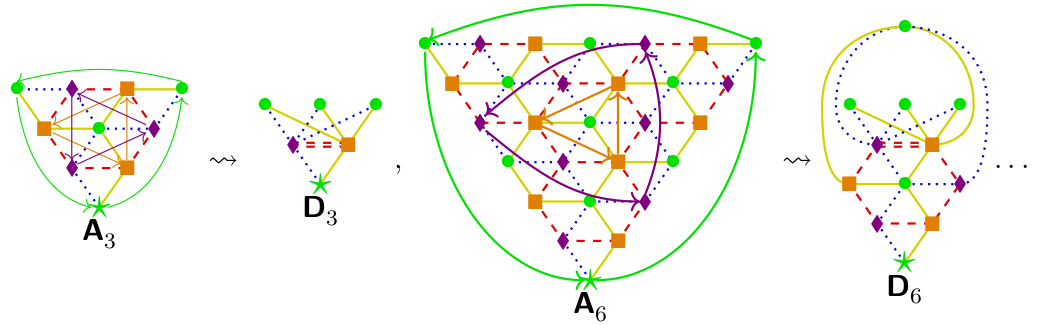## Data

• Title: Trihedral Soergel bimodules
• Authors: Marco Mackaay, Volodymyr Mazorchuk, Vanessa Miemietz and Daniel Tubbenhauer
• Status: Fund. Math. 248 (2020), no. 3, 219-300. Last update: Wed, 19 Jun 2019 06:17:53 UTC
• ArXiv version = 0.99 published version
• LaTex Beamer presentation: Slides1, Slides2 and Part II by Marco Mackaay: Slides, Slides3, Slides4, Slides5, Slides6

## Abstract

The quantum Satake correspondence relates dihedral Soergel bimodules to the semisimple quotient of the quantum $$\mathfrak{sl}_2$$ representation category. It also establishes a precise relation between the simple transitive 2-representations of both monoidal categories, which are indexed by bicolored $$\mathsf{ADE}$$ Dynkin diagrams.
Using the quantum Satake correspondence between affine $$\mathsf{A}_{2}$$ Soergel bimodules and the semisimple quotient of the quantum $$\mathfrak{sl}_3$$ representation category, we introduce trihedral Hecke algebras and Soergel bimodules, generalizing dihedral Hecke algebras and Soergel bimodules. These have their own Kazhdan-Lusztig combinatorics, simple transitive 2-representations corresponding to tricolored generalized $$\mathsf{ADE}$$ Dynkin diagrams.

## A few extra words

In pioneering work, Kazhdan-Lusztig defined their celebrated bases of Hecke algebras. Crucially, on these bases the structure constants of the algebras belong to $$\mathbb{N}_0=\mathbb{Z}_{\geq 0}$$. This started a program to study $$\mathbb{N}_0$$-algebras, which have a fixed basis with non-negative integral structure constants.
For these algebras it makes sense to study and classify $$\mathbb{N}_0$$-representations, i.e. representations with a fixed basis on which the fixed bases elements of the algebra act by non-negative integral matrices. As it turns out, $$\mathbb{N}_0$$-representations are interesting from various points of view, with applications and connections to e.g. graph theory, conformal field theory, modular tensor categories and subfactor theory.
The categorical incarnation of $$\mathbb{N}_0$$-representation theory is 2-representation theory, with simple transitive 2-representations playing the role of the simple representations.
In this paper, we restrict our attention to certain algebras which we call trihedral Hecke algebras, generalizing dihedral Hecke algebras, and their categorification by trihedral Soergel bimodules, generalizing dihedral Soergel bimodules.
The analogy of the trihedral to the dihedral case is as follows, where we always exclude the (trivial) cases of $$\mathbb{N}_0$$-representations or 2-representations of rank one:

Dihedral Trihedral
The dihedral Hecke algebra is a quotient of the affine type $$\mathsf{A}_1$$ Hecke algebra. The trihedral Hecke algebra is a subquotient of the affine type $$\mathsf{A}_2$$ Hecke algebra.
All simple representations are two dimensional. All simple representations are three dimensional.
All simple representations can be constructed by using the roots of the Chebyshev polynomials. All simple representations can be constructed by using the roots of Koornwinder's two variable version of the Chebyshev polynomials.
The $$\mathbb{N}_0$$-representations are given by bicolored $$\mathsf{ADE}$$ Dynkin diagrams. The $$\mathbb{N}_0$$-representations should be classified by tricolored generalized $$\mathsf{ADE}$$ Dynkin diagrams.
The simple transitive 2-representations are classified by bicolored $$\mathsf{ADE}$$ Dynkin diagrams. The simple transitive 2-representations should be classified by tricolored generalized $$\mathsf{ADE}$$ Dynkin diagrams.
The quiver algebras underlying the simple transitive 2-representations of rank greater than one are the zigzag algebras of the corresponding Dynkin type. The quiver algebras underlying the simple transitive 2-representations of rank greater than one should be analogs zigzag algebras of the corresponding generalized Dynkin type.
Here is an example how the generalized generalized $$\mathsf{ADE}$$ Dynkin diagrams look like:The type $$\mathsf{A}$$ family is supported on a cut-off of the $$\mathfrak{sl}_3$$ weight lattices, similarly as usual type $$\mathsf{A}$$ Dynkin diagrams are supported on cut-offs of the $$\mathfrak{sl}_2$$ weight lattices. The type $$\mathsf{D}$$ family comes from a $$\mathbb{Z}/3\mathbb{Z}$$ symmetry from the type $$\mathsf{A}$$ family, similarly as the type $$\mathsf{D}$$ Dynkin diagrams come from a $$\mathbb{Z}/2\mathbb{Z}$$ symmetry from the type $$\mathsf{A}$$ Dynkin diagrams.